[BACK]
 Computers, Materials & ContinuaDOI:10.32604/cmc.2022.022458Article

Denoising Letter Images from Scanned Invoices Using Stacked Autoencoders

1Department of Information Technology, College of Computer and Information Sciences, Princess Nourah bint Abdulrahman University, Riyadh, 84428, Saudi Arabia
2Department of Electronics, College of Engineering Chengannur, Kerala Technological University, Chengannur, 689121, India
*Corresponding Author: Samah Ibrahim Alshathri. Email: sealshathry@pnu.edu.sa
Received: 08 August 2021; Accepted: 09 September 2021

Abstract: Invoice document digitization is crucial for efficient management in industries. The scanned invoice image is often noisy due to various reasons. This affects the OCR (optical character recognition) detection accuracy. In this paper, letter data obtained from images of invoices are denoised using a modified autoencoder based deep learning method. A stacked denoising autoencoder (SDAE) is implemented with two hidden layers each in encoder network and decoder network. In order to capture the most salient features of training samples, a undercomplete autoencoder is designed with non-linear encoder and decoder function. This autoencoder is regularized for denoising application using a combined loss function which considers both mean square error and binary cross entropy. A dataset consisting of 59,119 letter images, which contains both English alphabets (upper and lower case) and numbers (0 to 9) is prepared from many scanned invoices images and windows true type (.ttf) files, are used for training the neural network. Performance is analyzed in terms of Signal to Noise Ratio (SNR), Peak Signal to Noise Ratio (PSNR), Structural Similarity Index (SSIM) and Universal Image Quality Index (UQI) and compared with other filtering techniques like Nonlocal Means filter, Anisotropic diffusion filter, Gaussian filters and Mean filters. Denoising performance of proposed SDAE is compared with existing SDAE with single loss function in terms of SNR and PSNR values. Results show the superior performance of proposed SDAE method.

Keywords: Stacked denoising autoencoder (SDAE); optical character recognition (OCR); signal to noise ratio (SNR); universal image quality index (UQ1) and structural similarity index (SSIM)

1  Introduction

Digitizing paper documents is a crucial step in business process automation. This process helps industries to efficiently manage large volume of documents. The images obtained by scanning the paper documents are converted into a digital format using OCR (optical character recognition) software. Usually, during the scanning process, noise can get into the images in the form of background noise, blurred and faded letters due to dirt on paper or lens, water marks, moisture on the lens, or due to physical dealing of papers. Transmission errors and compression methods also add noise to the images . This can result in significant image degradation and affects OCR detection accuracy. So, it is essential to use efficient image denoising techniques as a preprocessing step in order to remove the noise and recover the text information from degraded image obtained. Invoice data is an important document that need to be automated in almost all industries. Most of the old invoice data(receipts) may be damaged due to physical handling and dirt. During scanning and detection process, OCR fail to detect accurately the letters in these noisy invoices. So invoice data denoising is required as a preprocessing step before OCR detection process. Most of the existing filtering methods are not efficient for this type of letter images at high noise conditions. This work focus on an autoencoder based deep learning technique for invoice letter image denoising. We prepared a dataset of 59,119 letter images obtained from different scanned invoice images and developed a modified stacked denoising autoencoder model with combined loss function criteria for letter denoising. A detailed comparison is made with existing denoising filters and standard autoencoder based method for different noise levels.

The image denoising techniques have attracted researchers for half a century and, it remains a challenging and open task . The Spatial domain methods consist of linear filters, which blurs edges and remove fine details  and non-linear filters, which preserve the edge information while suppressing noise . Denoising filters in literature consists of wiener filtering technique, morphological techniques, vector-median-filtering, non-local algorithm method etc. . But these methods cannot produce better results for document images.

Machine learning methods for image denoising includes, sparse based methods, dictionary learning method, total variation regularization, gradient histogram estimation and preservation (GHEP) etc. . These methods have reasonably good performance, but have many drawbacks , such as manual setting of parameters and the need for high computational optimization techniques.

Deep learning techniques are part of machine learning, which have significant applications in many fields . Application of deep learning in image denoising have gained much attention in recent years . But in case of image denoising, most deep learning methods are highly data dependent and one architecture designed to remove a particular noise will not work for another type of noise distribution. To perform deep learning based denoising for invoice data, a large training dataset is required. No such public data for invoice letters is now available and this hinders research in this direction.

In this paper, a modified stacked denoising autoencoder is implemented and used for receipt data denoising. The proposed method of autoencoder design can capture the most salient features of training samples. An undercomplete autoencoder is designed with non-linear encoder and decoder function. This autoencoder is regularized for denoising application using a combined loss function which considers both mean square error and binary cross entropy error. A two-level stacking is done to increase efficiency of the network. A dataset consisting of 59,119 letter images, is prepared from different scanned invoices(receipts) images and windows true type (.ttf) files for training the network. Its performance is compared with other denoising filters, in terms of SSIM index, SNR (dB), PSNR (dB) and UQI values for different noise levels. Denoising performance of proposed SDAE with combined loss function criteria is compared with standard SDAE with single loss function criteria in terms of SNR and PSNR values. Results shows that proposed method have better denoising performance.

2  Autoencoders

Autoencoders  are artificial neural networks which are capable of learning the lower-dimensional features of the input data. It is based on unsupervised training criterion. The input is in the form of a vector, which is represented as p ∈ [0, 1] d, which can be a patch of an image. This input is mapped to a hidden representation q ∈ [0, 1] d. Here ‘d’ represents the dimensionality of the vector space. The mapping is given by the Eq. (1)

q=s(Wp+b) (1)

where s is a nonlinear function. W is a weight matrix and b a bias vector. This hidden representation is mapped back to a vector y ∈ [0, 1] d, in order to obtain the reconstructed input data. This reverse mapping is given by the Eq. (2)

y=s(Wq+b) (2)

W′ and b′ are weight and bias of the network respectively.

The model parameters are optimized to minimize cost function, which is the average reconstruction error,

given by Eq. (3)

W,b,W,b=minW,b,W,b1ni=0nL(p(i),y(i)) (3)

where L is a loss function.

This network adapts itself to extract features from images. So hand coded feature descriptors are not needed. Autoencoder can be used for classification and denoising applications. Autoencoder architecture is shown in Fig. 1. Autoencoder consists of three layers, an encoder, one hidden layer and a decoder. The aim of an encoder is to take an input vector (p) and produce a feature map (q). This is a compressed representation of input data. The decoder reconstructs the output vector (y). During each training phase a loss function is calculated and its value is minimized, so that the reconstructed data looks like the original input.

2.1 Denoising Autoencoders

Denoising Autoencoder  can learn to remove noise from the input image. It can prevent overfitting in classification tasks, by preventing the network from memorizing examples from training set. For denoising purposes, instead of using the input and the reconstructed output to compute the loss, the loss can be calculated by using the ground truth image and the reconstructed image as shown in Fig. 2.

The mapping function for denoising autoencoder is given by the Eq. (4)

q=s(W(p+r)+b) (4)

where r is a random vector and s is a nonlinear function. This reverse mapping for denoising autoencoder is given by the Eq. (5)

y=s(Wq+b) (5)

W′ and b′ are weight and bias of the network respectively. The cost function is

J(W)=0.5Nn=0N(||p(n)y(n)||)+0.5 Tr (WWT+W'TW') (6)

The second term in cost function is used to minimize correlations between input images.Figure 1: Autoencoder architecture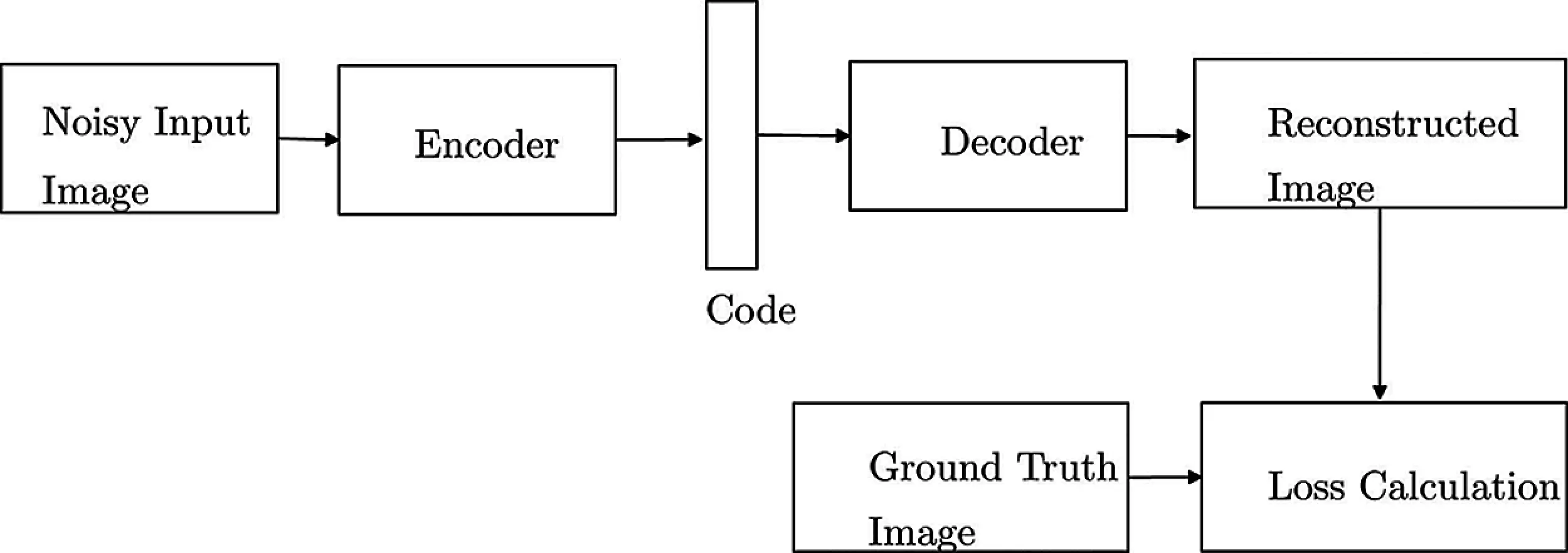Figure 2: Image denoising autoencoder

2.2 Stacked Autoencoders

Stacked Autoencoders is obtained by stacking one layer of autoencoder after the other . A composition of several levels of nonlinearity in a neural network can efficiently model complex relationships between variables. Each layer produces a higher-level representation from the lower-level representation. output by the previous layer. This technique can efficiently detect important structures(features) in the input patterns. A new encoding function is learnt by the network in each hidden layer and passed to next level for learning another encoding function. The structure of stacked autoencoder is shown in Fig. 3.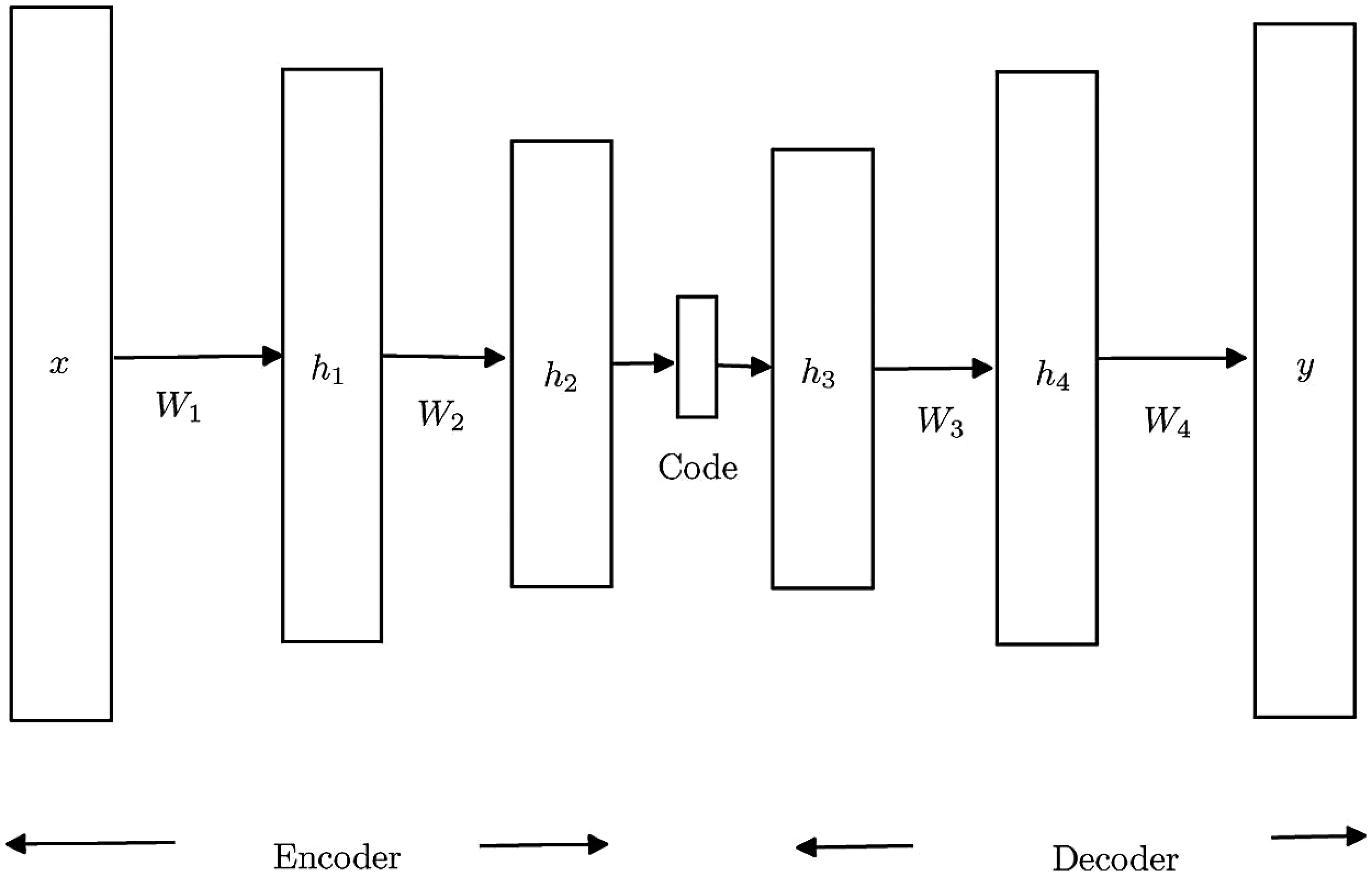Figure 3: Stacked autoencoder

Input is a vector x, which is passed through hidden layers and y is the decoded vector. Encoder is used for mapping the input data x into hidden representation (code), and decoder is used for reconstructing input data from the hidden representation. Here h1 (first hidden layer) represents the hidden encoder vector calculated from x and h2 (second hidden layer) represents the second hidden encoder vector calculated from layer h1. Similarly h3 and h4 are two hidden layers in the decoder section, which represents the hidden decoded vectors formed from the code generated by encoder. Here y is the decoded vector of the output layer. The encoding process in each layer is as follows:

hn=f(Wnxn+bn) (7)

where hn represents hidden encoder vector in nth hidden layer, f is the encoding function, Wn represents weight matrix of encoder in nth hidden layer, and bn is the bias vector in nth hidden layer.

hn=g(Wnxn+bn) (8)

where hn represents hidden decoder vector in nth hidden layer, g is the decoding function, Wn represents weight matrix of decoder in nth hidden layer, and bn is the bias vector in nth decoder hidden layer.

End to end pre-training and Ladder wise pre-training are the two methods of training stacked autoencoders. After all the hidden layers are trained, backpropagation algorithm is used to minimize the cost function and update the weights by optimization process. The rectified linear units (Re-LU) activation function is used after each hidden layer vector calculation. Re-LU does not suffer from gradient diffusion or vanishing problems. The Re-LU function is

fr(x)=max(0,x) (9)

Sigmoid activation function is used in output layer.

3  Methodology

Methodology of work is shown in Fig. 4. First the dataset for training/testing/cross validation is generated. An autoencoder is designed and the data set is used to train it. The performance of the autoencoder to remove additive noise is tested with external noisy letter images.

.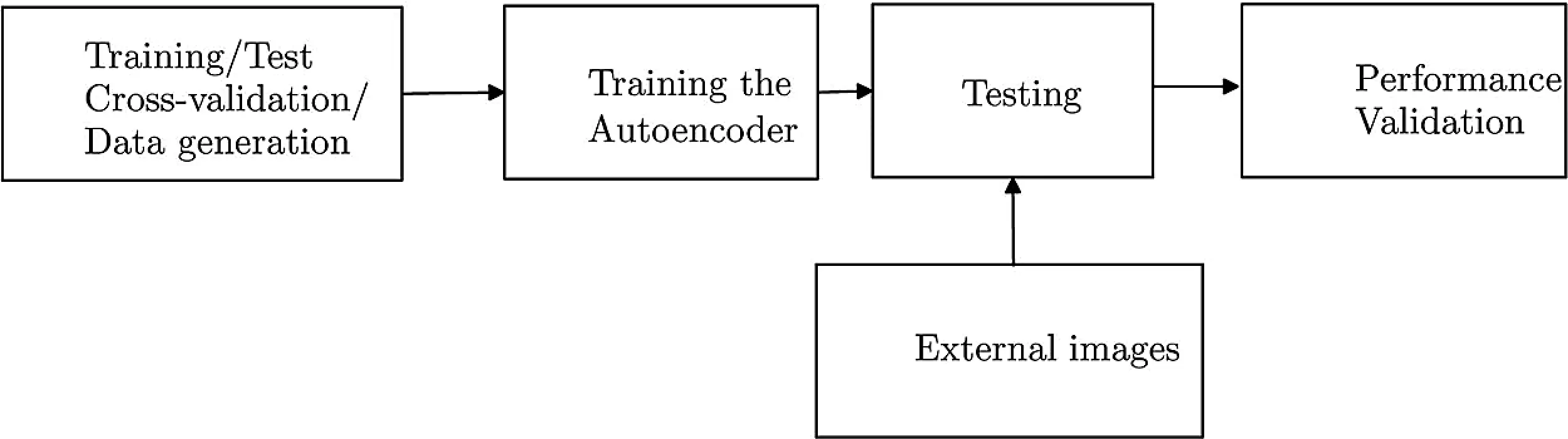Figure 4: Methodology of work

4  Experiment

The steps in the experiment are detailed in Fig. 5. This section is broadly divided into generation of dataset, development of stacked denoising autoencoder and testing.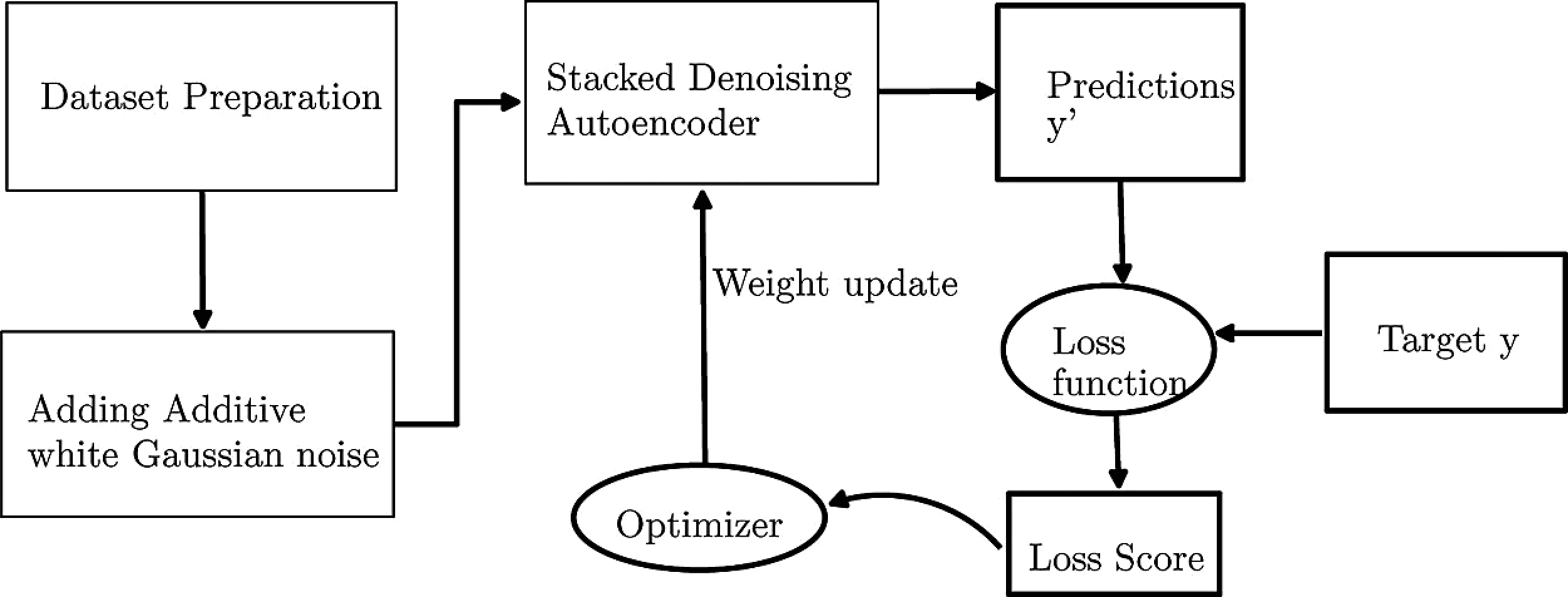Figure 5: Work flow

4.1 Generation of Data Set

Invoices are often generated by Windows based system and it is logical to train the autoencoder with Windows fonts and letter sizes. A Python script is written to extract Windows true type (.ttf) files and are used to generate images of lower case and upper case English letters and numerals. All possible fonts at 12 point size are used to generate synthetic images of dimension 60 × 40. The 62 letters and numbers are stored in 62 folders, each folder containing 711 images, with label as the folder name. This synthetic dataset contains 44082 images.

Another Python script is written to read in scanned images of invoices and contours are drawn around letters and these text boxes are separated, labelled and added to the respective folders to augment the synthetic data set to yield 59,119 images. The pixel values of the images are converted to a Python array along with the labels, added controlled amount of noise and then pickled to form the training, test and cross validation data for the autoencoder.

4.2 Development of Autoencoder

Stacked denoising autoencoder is implemented in python using Py-Torch deep learning library. Pickled noisy images of size 59,119 × 60 × 40 is fed to the input of stacked denoising autoencoder. Adam optimizer is used. Learning rate used is 10−3 and batch size used is 16. The network is trained for 100 epochs on an HPCC with NVIDIA Tesla k20M GPU hardware.

During each epoch, mean square error and cross entropy error are calculated and this loss score is backpropagated through an optimizer in order to update the weights of the network. Two hidden layers having 512 neurons and 128 neurons respectively are included in encoder section. Another two hidden layers having 128 neurons and 512 neurons respectively are included in decoder section. Re-Lu activation function is used after each hidden layer. Sigmoid activation function is used at the output layer. Additive Gaussian noise of mean zero and variance of 20% of the peak signal value is used to get the noisy version of letter images.

4.3 Testing

The system shown in Fig. 6 is tested with noisy letter images of known variance. End to end pre-training is done using 59,119 noisy letter images. A comparative study with other filters is done in terms of Peak Signal to Noise Ratio (PSNR), Signal to Noise Ratio (SNR), Structural Similarity Index (SSIM) and Universal Image Quality Index (UQI).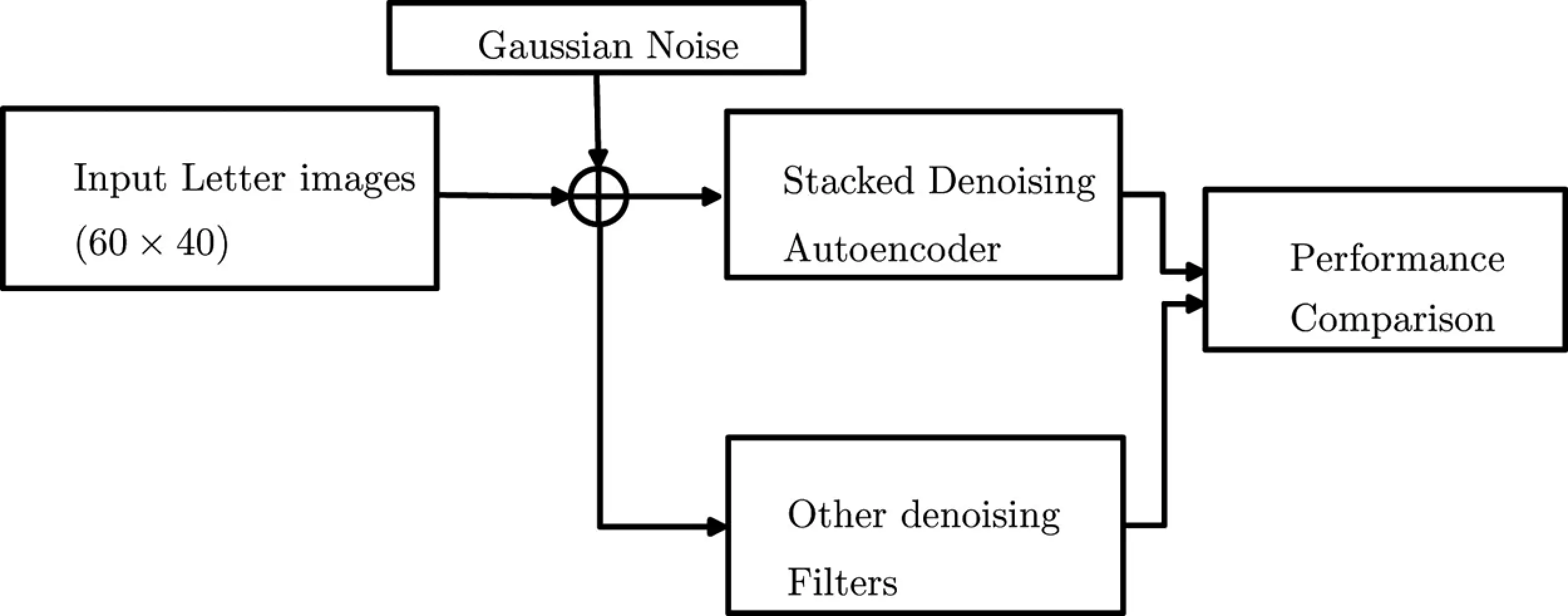Figure 6: Experimental setup for performance comparison

5  Results and Analysis

SDAE (Stacked denoising autoencoder) outputs with combined loss function for a randomly selected letter images for 20%, 40% and 60% (of the peak signal value)) noise variances are shown in Fig. 7. Observe the removal of noise in all cases. Here a letter “C” from scanned invoice, corrupted with 20% to 60% white gaussian noise is given as input and noise is perfectly removed in all cases. Python3 plotting library matplotlib is used for plotting all graphs and figures.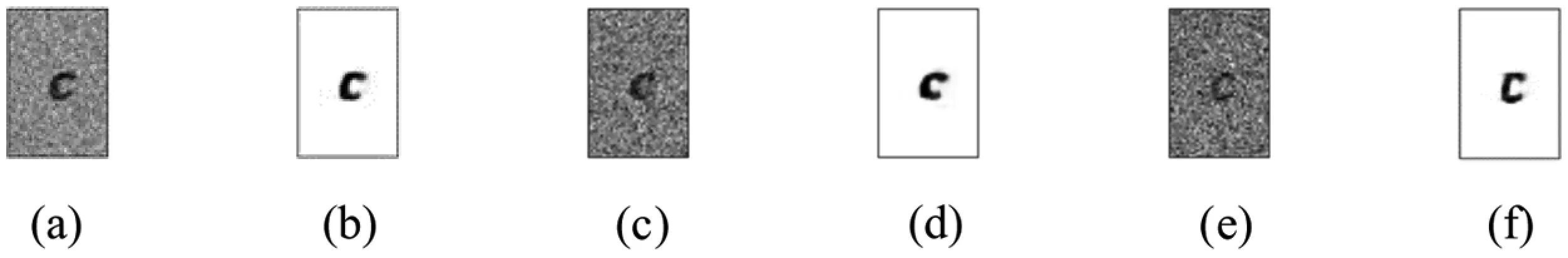Figure 7: Output of SDAE for different percentage of noise levels: (a) Image with 20% noise variance (b) Output of SDAE at 20% noise (c) Image with 40% noise variance (d) Output of SDAE at 40% noise (e) Image with 60% noise variance (f) Output of SDAE at 60% noise

Autoencoder output for a set of input letter images corrupted by 20% noise variance is shown in Fig. 8. Letters “y, m, M, 8, o, 7, h and 3” with 20% noise is tested. All images were denoised with 100% detection accuracy.Figure 8: First row shows original images, second row shows images with 20% noise variance and third row shows the SDAE output

Autoencoder output for the same set of input letter images corrupted by 40% noise variance is shown in Fig. 9. It is evident from figure that most letters were denoised perfectly. But number “3” does not retained its shape at this level of noise.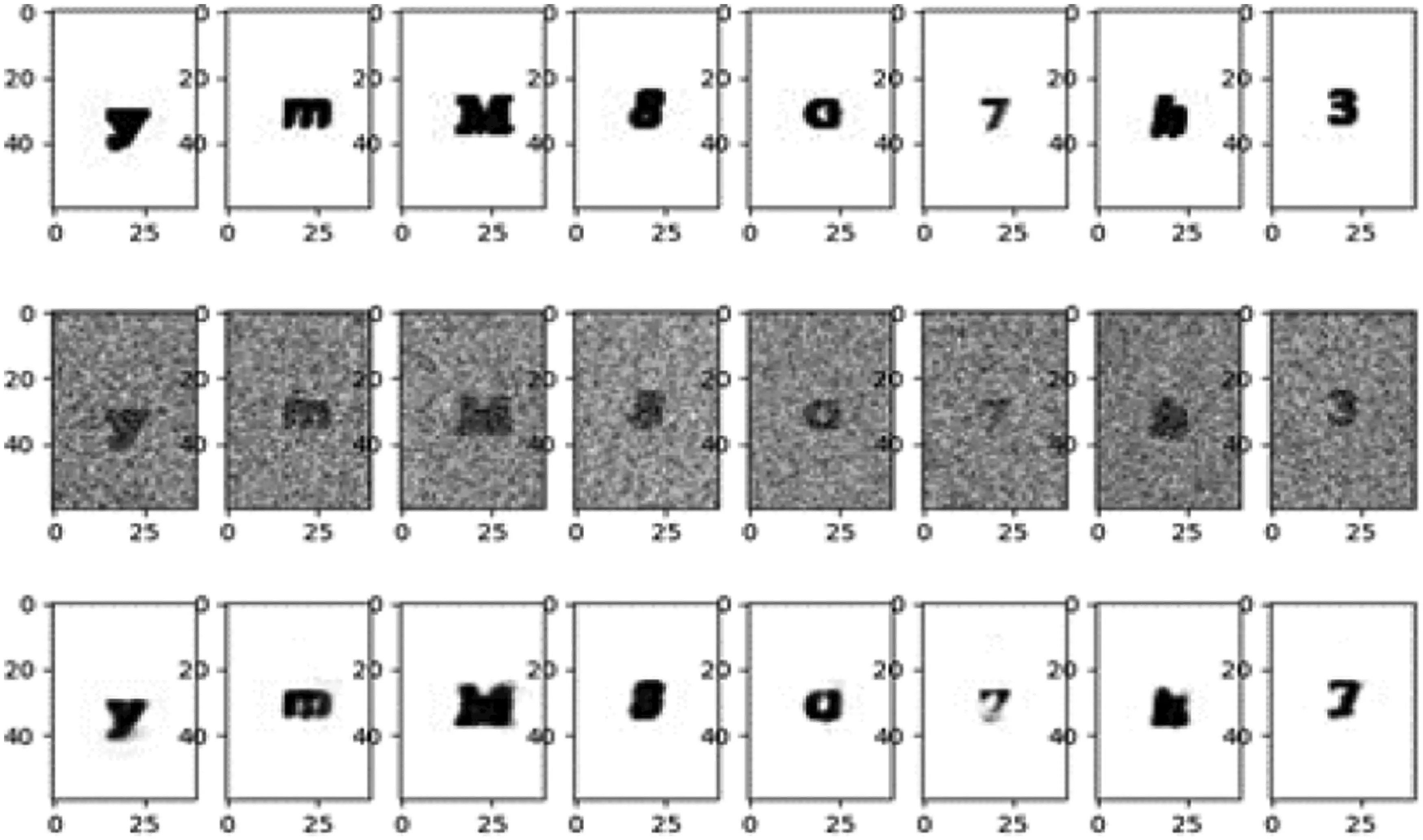Figure 9: First row shows original images, second row shows images with 40% noise variance and third row shows the SDAE output

Autoencoder output for Input letter images corrupted by 60% noise variance is shown in Fig. 10. It is observed that the proposed method works well under even deep noise levels. It is observed that the proposed method works well under even deep noise levels. Complete noise is removed and letters looks almost similar to input data.Figure 10: First row shows original images, second row shows images with 60% noise variance and third row shows the SDAE output

5.1 Comparison with Other Denoising Filters

It is essential to compare the performance of other denoising filters for these letter images from invoice at different noise levels. Results of other filters for a randomly selected invoice image representing number “4” corrupted by 20% noise variance are shown in Fig. 11 for comparison. NLM filter shows good denoising at this noise level. But the performance of anisotropic filter and gaussian filter are poor. SDAE removes complete noise and outperforms all other filters.Figure 11: Output of various filters for a letter image corrupted by 20% noise variance (a) Image with 20% noise variance (b) Output of SDAE (c) Output of anisotropic diffusion filter (d) Output of gaussian filter (e) Output of mean filter (f) Output of non-local means filter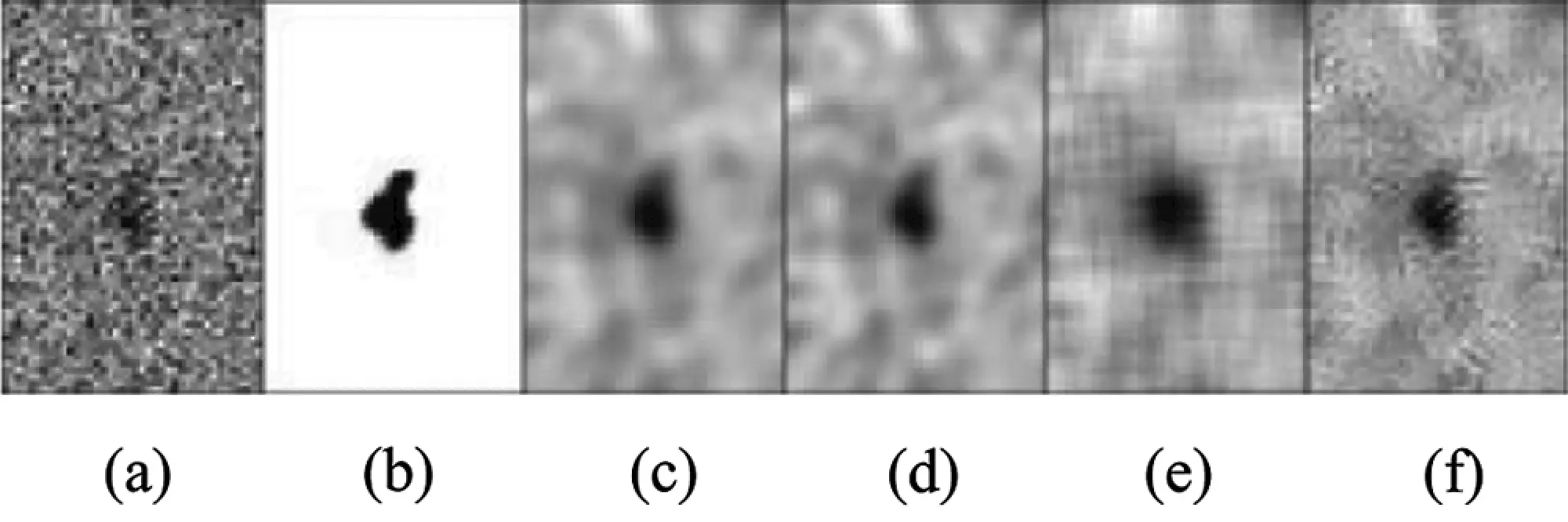Figure 12: Output of various filters for a letter image corrupted by 60% noise variance. (a) Image with 60% noise variance (b) Output of SDAE (c) Output of anisotropic diffusion filter (d) Output of gaussian filter (e) Output of mean filter (f) Output of non-local means filter

Results of other filters for the same image corrupted by 60% percentage of noise is shown Fig. 12. At this noise level no information is visible from noisy input image. SDAE detects letter “4” shows stable performance. NLM based method also fails at this level. At this high noise level, no other filters works better than SDAE.

Visual quality of SDAE is determined based on

1.    Signal to Noise Ratio (SNR)

2.    Peak Signal to Noise Ratio (PSNR)

3.    Structural Similarity Index (SSIM)

4.    Universal Image Quality Index (UQI)

5.1.1 Improvement in Signal to Noise Ratio

The SNR is expressed as

SNR=10log10n1n2r[n1,n2]2n1n2[r[n1,n2]2t[n1,n2]2] (10)

Signal to noise ratio improvement of various denoising methods for Gaussian noise of zero mean and different noise variances is shown in Tab. 1. It is observed that the SNR improvement for SDAE is consistently above other filters by 10–12 dB even under deep noise, validating the visual quality in Figs. 11 and 12. But the visual quality of NLM filter, Anisotropic diffusion and Gaussian Filter are not stable with high noise variances. This is evident from SSIM values.

5.1.2 Peak Signal to Noise Ratio

Peak signal to noise ratio (PSNR) is the ratio between the maximum possible power of an image and the power of corrupting noise that affects the quality of its representation. PSNR is defined as follows:

PSNR =20log10[M1RMSE] (11)

Here, M is the number of maximum possible intensity levels (minimum intensity level considered to be 0) in an image and RMSE is root mean square error. Tab. 2 shows the PSNR values for various filter under different noise levels. The values for SDAE are above other filters, indicating its superior performance in noise removal.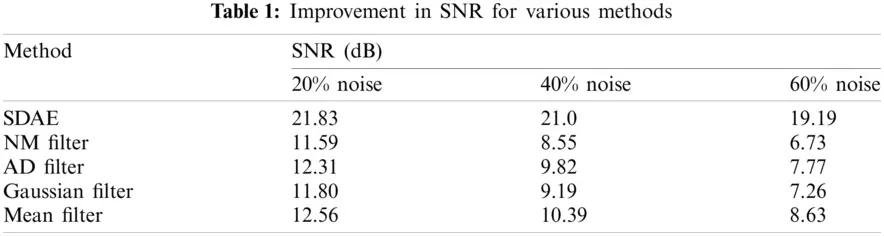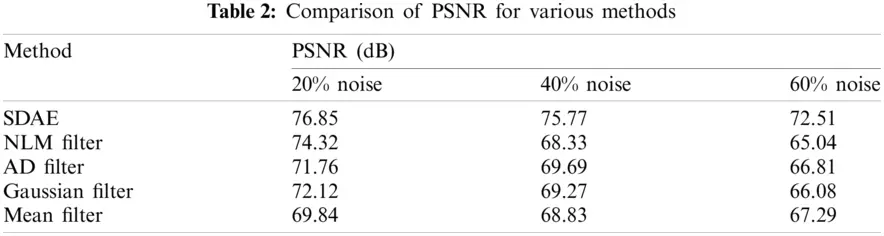5.1.3 Structural Similarity Index (SSIM)

Structural similarity index (SSIM)  represents the “visual quality” of the image. It quantifies the degree of preservation of the overall structure of the image. The similarity index between the images x and y is given as

SSIM=(2μxμy+C1)(2σxy+C2)(2μX2+2μY2+C1)(2σX2+2σY2+C2) (12)

The parameters μx and μy are the means and σx2 and σy2 are the variances of x and y respectively. σxy is the covariance between x and y. C1 and C2 are nonzero constants. When x and y are identical, SSIM is unity and degrades when the structural differences between x and y increases.

SSIM comparison of five denoising methods, SDAE, NLM, Gaussian filter, Mean filter and anisotropic diffusion filter is shown in Fig. 13. The stable performance of SDAE can be accounted from this graph. At 10% of gaussian noise, NLM is having slightly higher SSIM index. But its performance drastically reduced with increased noise levels. Anisotropic diffusion filter shows a stable value, but its SSIM value is less than SDAE. Higher value in the range of 0.998 is obtained from SDAE. Gaussian and mean filters shows lower values of SSIM.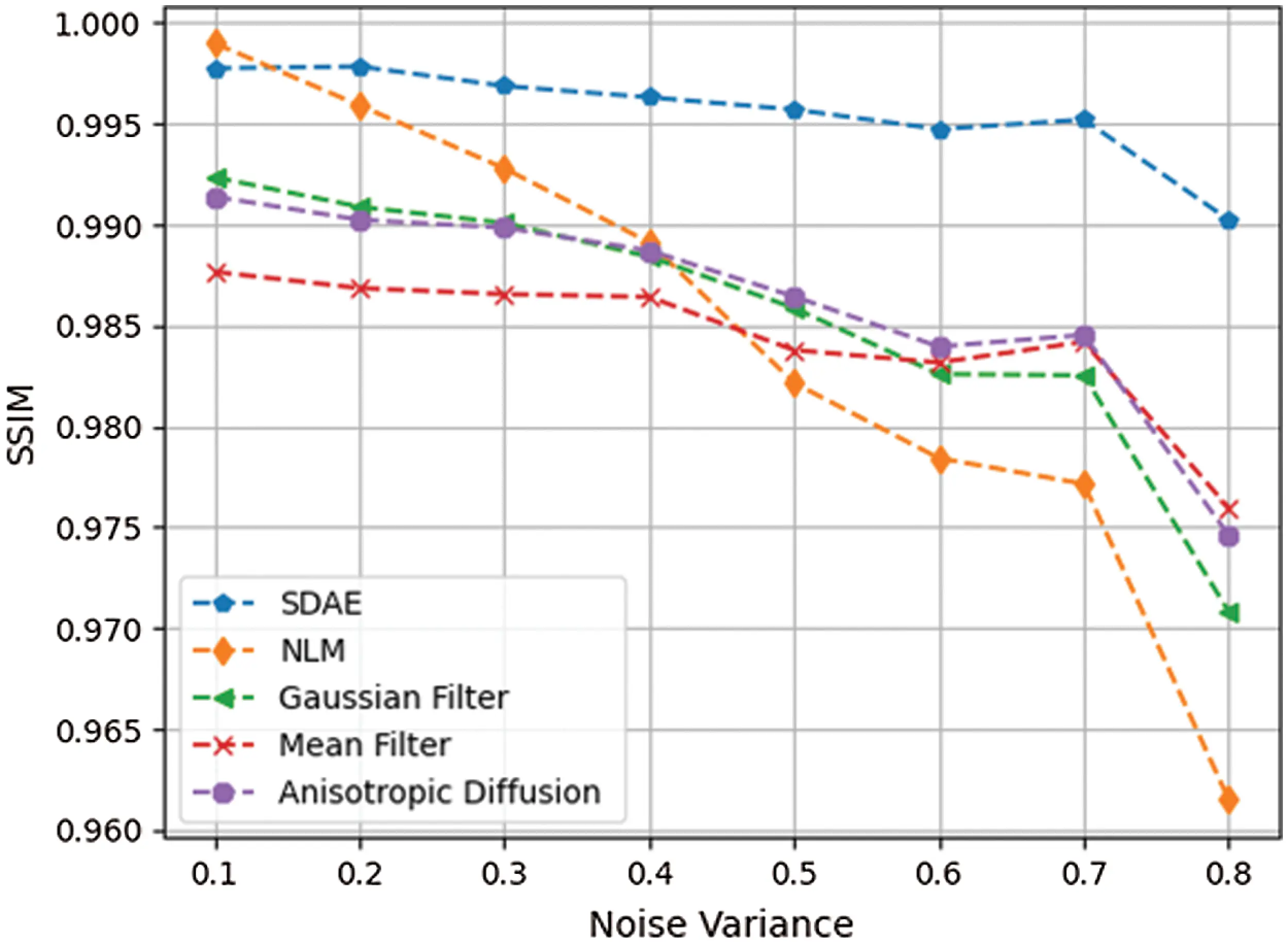Figure 13: SSIM comparison of five different methods

5.1.4 Universal Image Quality Index (UQI)

UQI  is designed by modelling any image distortion as a combination of three factors: loss of correlation, luminance distortion, and contrast distortion. Comparison of Universal image Quality Index for different methods is shown in Fig. 14. NLM filter have good performance only for low noise levels SDAE shows a stable and better Universal image Quality Index even for high noise levels. Gaussian filter has UQI values better than anisotropic diffusion filters for lower noise levels. But stability of gaussian filter is less compared to anisotropic diffusion method. Mean filter has lowest UQI values.

5.2 Comparison with Standard SDAE

The proposed stacked denoising autoencoder with combined MSE and BCE loss function is compared with standard stacked denoising autoencoder with single binary cross entropy loss function, in terms of signal to noise ratio (SNR) and peak signal to noise ratio (PSNR). Comparison results for two different noise levels for a single selected letter ‘X’ are shown in Tab. 3. Results shows that proposed method have good denoising capability even at higher noise levels.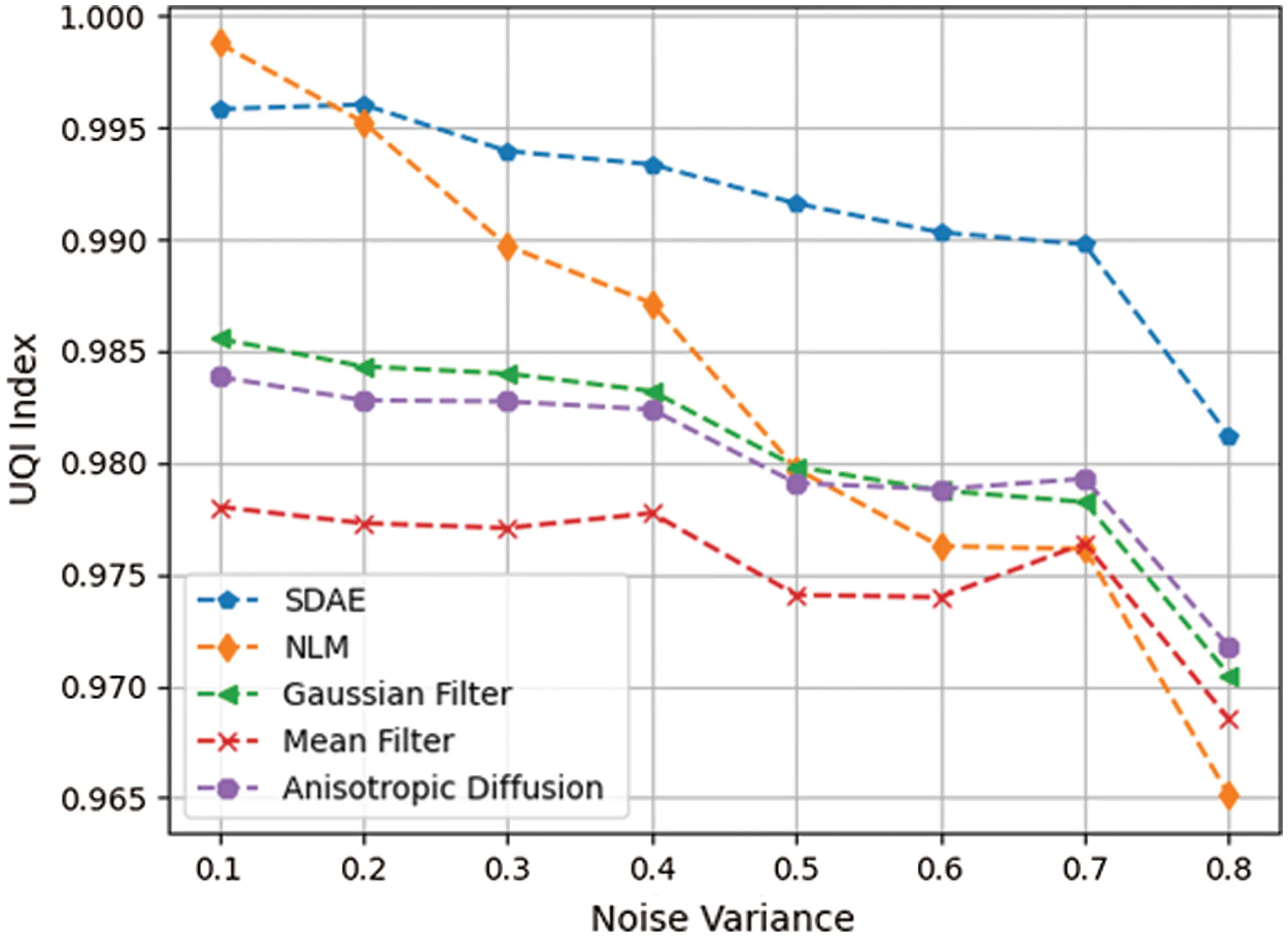Figure 14: Comparison of universal image quality index for different methods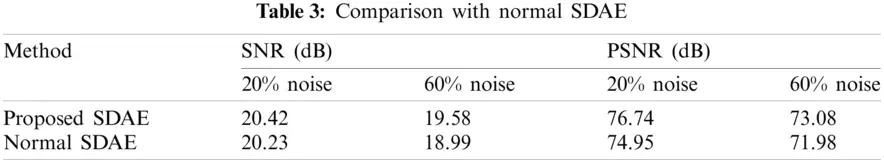6  Conclusion

The proposed denoising method of letter images from invoice documents by using modified Stacked Denoising Autoencoder (SDAE) is observed to have excellent signal to noise ratio, structural similarity index and universal quality index, even under extreme noisy conditions. Using a combined loss function which considers both mean square error and binary cross entropy for regularizing the denoising function is used. Under complete representation of autoencoder used in this denoising method have better feature extraction properties. A dataset consisting of 59,119 letter images, which contains both English alphabets (upper and lower case) and numbers (0 to 9) is prepared from many scanned invoices images and windows true type (.ttf) files and is used for training the neural network. SDAE being an unsupervised deep learning method, no labels are required for training the network. No manual parameter tuning is necessary when compared to other denoising filters. The denoised letters have better chances of detection by OCR methods. SDAE denoising performance in terms of SNR, PSNR, SSIM and UQI values is compared with non-local means filter, anisotropic diffusion filter, gaussian filter and mean filters. The proposed SDAE method is also compared with standard SDAE in terms of SNR and PSNR values. A SSIM value as high as 0.998912 is obtained irrespective of extreme noise levels. One disadvantage is the large time taken for training the network. Once the model is saved, it can be reused. Another disadvantage is due to limitation in no of training samples of some letters from 62 different classes, its shape may get deformed at extreme noise levels. These issues may be addressed in future research. Also new regularization and optimization methods can be incorporated to increase the denoising performance.

Acknowledgement: This research was funded by the Deanship of Scientific Research at Princess Nourah bint Abdulrahman University through the Fast-track Research Funding Program.

Funding Statement: This research was funded by the Deanship of Scientific Research at Princess Nourah bint Abdulrahman University through the Fast-track Research Funding Program.

Conflicts of Interest: The authors declare that they have no conflicts of interest to report regarding the present study

## References

1. J. Xu, L. Zhang and D. Zhang, “External prior guided internal prior learning for real-world noisy image denoising,” IEEE Transactions on Image Processing, vol. 27, no. 6, pp. 2996–3010, 2018.
2. L. Fan, F. Zhang, H. Fan and C. Zhang, “Brief review of image denoising techniques,” Visual Computing for Industry, Biomedicine, and Art, vol. 2, no. 1, pp. 1–12, 2019.
3. P. Milanfar, “A tour of modern image filtering: New insights and methods, both practical and theoretical,” IEEE Signal Processing Magazine, vol. 30, no. 1, pp. 106–128, 2012.
4. M. C. Motwani, M. C. Gadiya, R. C. Motwani and F. C. Harris, “Survey of image denoising techniques,” in Proc. of Global Signal Processing Expo Conf. (GSPXUSA, pp. 27–30, 200
5. P. Jain and V. Tyagi, “A survey of edge-preserving image denoising methods,” Information Systems Frontiers, vol. 18, no. 1, pp. 159–170, 2016.
6. L. Fan, L. Fan and C. L. Tan, “Binarizing document image using coplanar prefilter,” in Proc. of Sixth IEEE Int. Conf. on Document Analysis and Recognition, Seattle, WA, USA, pp. 34–38, 2001.
7. I. Pitas and A. Venetsanopoulos, “Nonlinear mean filters in image processing,” IEEE Transactions on Acoustics, Speech, and Signal Processing, vol. 34, no. 3, pp. 573–584, 1986.
8. H. Farahanirad, J. Shanbehzadeh, M. M. Pedram and A. Sarrafzadeh, “A hybrid edge detection algorithm for salt-and-pepper noise,” in Proc. of the Int. Multi Conf. of Engineers and Computer Scientists (IMCECHong Kong, pp. 475–479, 2011.
9. H. S. M. Al-Khaffaf, A. Z. Talib and R. A. Salam, “Removing salt-and-pepper noise from binary images of engineering drawings,” in Proc. of 19th IEEE Int. Conf. on Pattern Recognition, Tampa, FL, USA, pp. 1–4, 2008.
10. M. Barni, F. Buti, F. Bartolini and V. Cappellini, “A quasi-euclidean norm to speed up vector median filtering,” IEEE Transactions on Image Processing, vol. 9, no. 10, pp. 1704–1709, 2000.
11. E. Abreu, M. Lightstone, S. K. Mitra and K. Arakawa, “A new efficient approach for the removal of impulse noise from highly corrupted images,” IEEE Transactions on Image Processing, vol. 5, no. 6, pp. 1012–1025, 1996.
12. Z. Wang and D. Zhang, “Progressive switching median filter for the removal of impulse noise from highly corrupted images,” IEEE Transactions on Circuits and Systems II: Analog and Digital Signal Processin, vol. 46, no. 1, pp. 78–80, 1999.
13. R. Bernstein, “Adaptive nonlinear filters for simultaneous removal of different kinds of noise in images,” IEEE Transactions on Circuits and Systems, vol. 34, no. 11, pp. 1275–1291, 1987.
14. V. S. Hari, V. P. J. Raj and R. Gopikakumari, “Unsharp masking using quadratic filter for the enhancement of fingerprints in noisy background,” Pattern Recognition, vol. 46, no. 12, pp. 3198–3207, 2013.
15. S. W. Hong and P. Bao, “An edge-preserving sub-band coding model based on non-adaptive and adaptive regularization,” Image and Vision Computing, vol. 18, no. 8, pp. 573–582, 2000.
16. A. Buades, B. Coll and J. M. Morel, “A review of image denoising algorithms, with a new one,” Multiscale Modeling & Simulation, vol. 4, no. 2, pp. 490–530, 2005.
17. T. Saba, A. Rehman, A. Al-Dhelaan and M. Al. Rodhaan, “Evaluation of current documents image denoising techniques: A comparative study,” Applied Artificial Intelligence, vol. 28, no. 9, pp. 879–887, 2014.
18.  D. Li, “Support vector regression based image denoising,” Image and Vision Computing, vol. 27, no. 6, pp. 623–627, 2009.
19. A. Buades, B. Coll and J. M. Morel, “A non-local algorithm for image denoising,” in 2005 IEEE Computer Society Conf. on Computer Vision and Pattern Recognition (CVPR’05San Diego, CA, USA, 2, pp. 60–65, 2005.
20. K. Dabov, A. Foi, V. Katkovnik and K. Egiazarian, “Image denoising by sparse 3-d transform-domain collaborative filtering,” IEEE Transactions on Image Processing, vol. 16, no. 8, pp. 2080–2095, 2007.
21. M. Elad and M. Aharon, “Image denoising via sparse and redundant representations over learned dictionaries,” IEEE Transactions on Image Processing, vol. 15, no. 12, pp. 3736–3745, 2006.
22. S. Osher, M. Burger, D. Goldfarb, J. Xu, W. Yin et al., “An iterative regularization method for total variation-based image restoration,” Multiscale Modeling & Simulation, vol. 4, no. 2, pp. 460–489, 2005.
23. U. Schmidt and S. Roth, “Shrinkage fields for effective image restoration,” in Proc. of the IEEE Conf. on Computer Vision and Pattern Recognition, Columbus, Ohio, pp. 2774–2781, 2014.
24. S. Gu, L. Zhang, W. Zuo and X. Feng, “Weighted nuclear norm minimization with application to image denoising,” in Proc. of the IEEE Conf. on Computer Vision and Pattern Recognition, Columbus, Ohio, pp. 2862–2869, 2014.
25. J. Mairal, F. Bach, J. Ponce, G. Sapiro and A. Zisserman, “Non-local sparse models for image restoration,” in 2009 IEEE 12th Int. Conf. on Computer Vision, Kyoto, Japan, pp. 2272–2279, 2009.
26. Y. Chen and T. Pock, “Trainable nonlinear reaction diffusion: A flexible framework for fast and effective image restoration,” IEEE Transactions on Pattern Analysis and Machine Intelligence, vol. 39, no. 6, pp. 1256–1272, 2016.
27. W. Zuo, L. Zhang, C. Song, D. Zhang and H. Gao, “Gradient histogram estimation and preservation for texture enhanced image denoising,” IEEE Transactions on Image Processing, vol. 23, no. 6, pp. 2459–2472, 2014.
28. G. Ongie, A. Jalal, C. A. Metzler, R. G. Baraniuk, A. G. Dimakis et al., “Deep learning techniques for inverse problems in imaging,” IEEE Journal on Selected Areas in Information Theory, vol. 1, no. 1, pp. 39–56, 2020.
29. M. Khayyat, I. A. Elgendy, A. Muthanna, A. S. Alshahrani, S. Alharbi et al., “Advanced deep learning-based computational offloading for multilevel vehicular edge-cloud computing networks,” IEEE Access, vol. 8, pp. 137052–137062, 2020.
30. C. Shoren, T. M. Khoshgoftaar and B. Furht, “Deep learning applications for COVID-19,” Journal of Big Data, vol. 8, no. 18, pp. 1–54, 2021.
31. I. A. Elgendy, W. Z. Zhang, H. He, B. B. Gupta and A. A. A. El-Latif, “Joint computation offloading and task caching for multi-user and multi-task MEC systems: Reinforcement learning-based algorithms,” Wireless Networks, vol. 27, no. 1, pp. 2023–2038, 2021.
32. M. Sit, B. Z. Demiray, Z. Xiang, G. J. Ewing, Y. Sermet et al., “A comprehensive review of deep learning applications in hydrology and water resources,” Water Science Technology, vol. 82, no. 12, pp. 2635–2670, 2020.
33. I. A. Elgendy, A. Muthanna, M. Hammoudeh, H. Shaiba, D. Unal et al., “Advanced deep learning for resource allocation and security aware data offloading in industrial mobile edge computing,” Journal of Big Data, vol. 9, no. 4, pp. 265–278, 2021.
34. A. Krizhevsky, I. Sutskever and G. E. Hinton, “Imagenet classification with deep convolutional neural networks,” Advances in Neural Information Processing Systems, vol. 25, pp. 1097–1105, 2012.
35. C. Tian, L. Fei, W. Zheng, Y. Xu, W. Zuo et al., “Deep learning on image denoising: An overview,” Neural Networks, vol. 131, no. 1, pp. 251–275, 2020.
36. Q. Xu, C. Zhang and L. Zhang, “Denoising convolutional neural network,” in IEEE Int. Conf. on Information and Automation, Lijiang, China, pp. 1184–1187, 2015.
37. V. Jain and S. Seung, “Natural image denoising with convolutional networks,” Advances in Neural Information Processing System, vol. 21, pp. 769–776, 2008.
38. P. Vincent, H. Larochelle, Y. Bengio and P. A. Manzagol, “Extracting and composing robust features with denoising autoencoders,” in Proc. of the 25th Int. Conf. on Machine Learning, Helsinki, Finland, pp. 1096–1103, 2008.
39. J. Liang and R. Liu, “Stacked denoising autoencoder and dropout together to prevent overfitting in deep neural network,” in 8th Int. Congress on Image and Signal Processing (CISPShenyang, China, pp. 697–701, 2015.
40. I. Goodfellow, Y. Bengio and A. Courville, Autoencoders. In: Deep Learning, 1st ed., vol. 1. London, U.K: MIT Press, pp. 496–506, 2016.
41. P. Vincent, H. Larochelle, I. Lajoie, Y. Bengio, P. A. Manzagol et al., “Stacked denoising autoencoders: Learning useful representations in a deep network with a local denoising criterion,” Journal of Machine Learning Research, vol. 11, no. 12, pp. 3371–3408, 2010.
42. Z. Wang, A. C. Bovik, H. R. Sheikh and E. P. Simoncelli, “Image quality assessment: From error visibility to structural similarity,” IEEE Transactions on Image Processing, vol. 13, no. 4, pp. 600–612, 2004.
43. Z. Wang and A. C. Bovik, “A universal image quality index,” IEEE Signal Processing Letters, vol. 9, no. 3, pp. 81–84, 2002.This work is licensed under a Creative Commons Attribution 4.0 International License, which permits unrestricted use, distribution, and reproduction in any medium, provided the original work is properly cited.